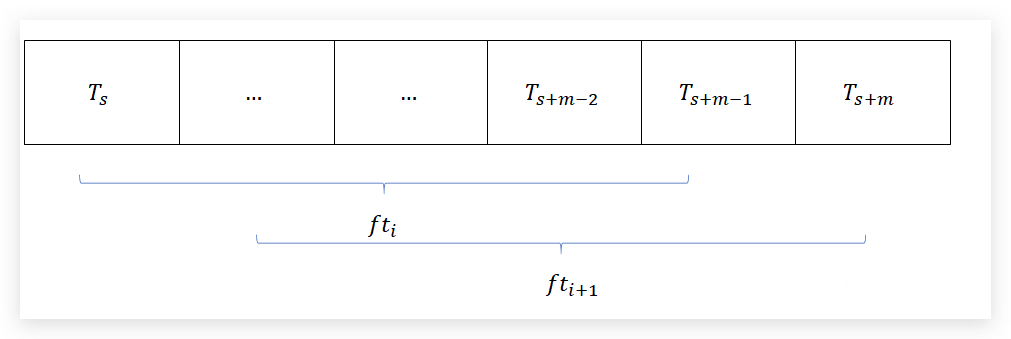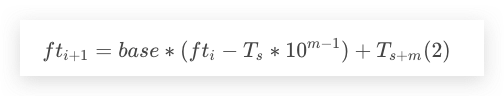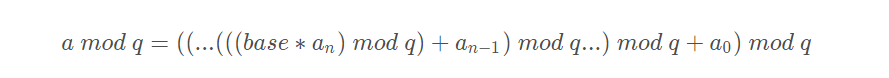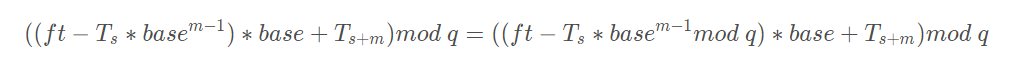Rabin Karp算法即是解决这个问题的更高效的一个算法。它的思想是，不直接逐位对比模式串pat和text是否相等，而是利用哈希算法，计算模式串和子串的哈希值，如果哈希值不相等，那么很明显字符串也不相等，如果相等，由于哈希算法可能会存在哈希冲突的可能，因此我们需要再使用朴素算法判断其是否真正相等。

num = a[n]*10^0 + a[n-1]*10^1 + a[n-2]*10^2 + ... + a*10^(n-1) + a*10^n

num = a[n]*26^0 + a[n-1]*26^1 + a[n-2]*26^2 + ... + a*26^(n-1) + a*26^n

hash1 = code(a)*26^1 + code(b)*26^0

hash2 = code(b)*26^1 + code(c)*26^0hash( txt[s+1 .. s+m] ) = ( d ( hash( txt[s .. s+m-1]) – txt[s]*h ) + txt[s + m] ) mod q
hash( txt[s .. s+m-1] ) : Hash value at shift s.
hash( txt[s+1 .. s+m] ) : Hash value at next shift (or shift s+1)
d: Number of characters in the alphabet
q: A prime number
h: d^(m-1)

Java代码实现：